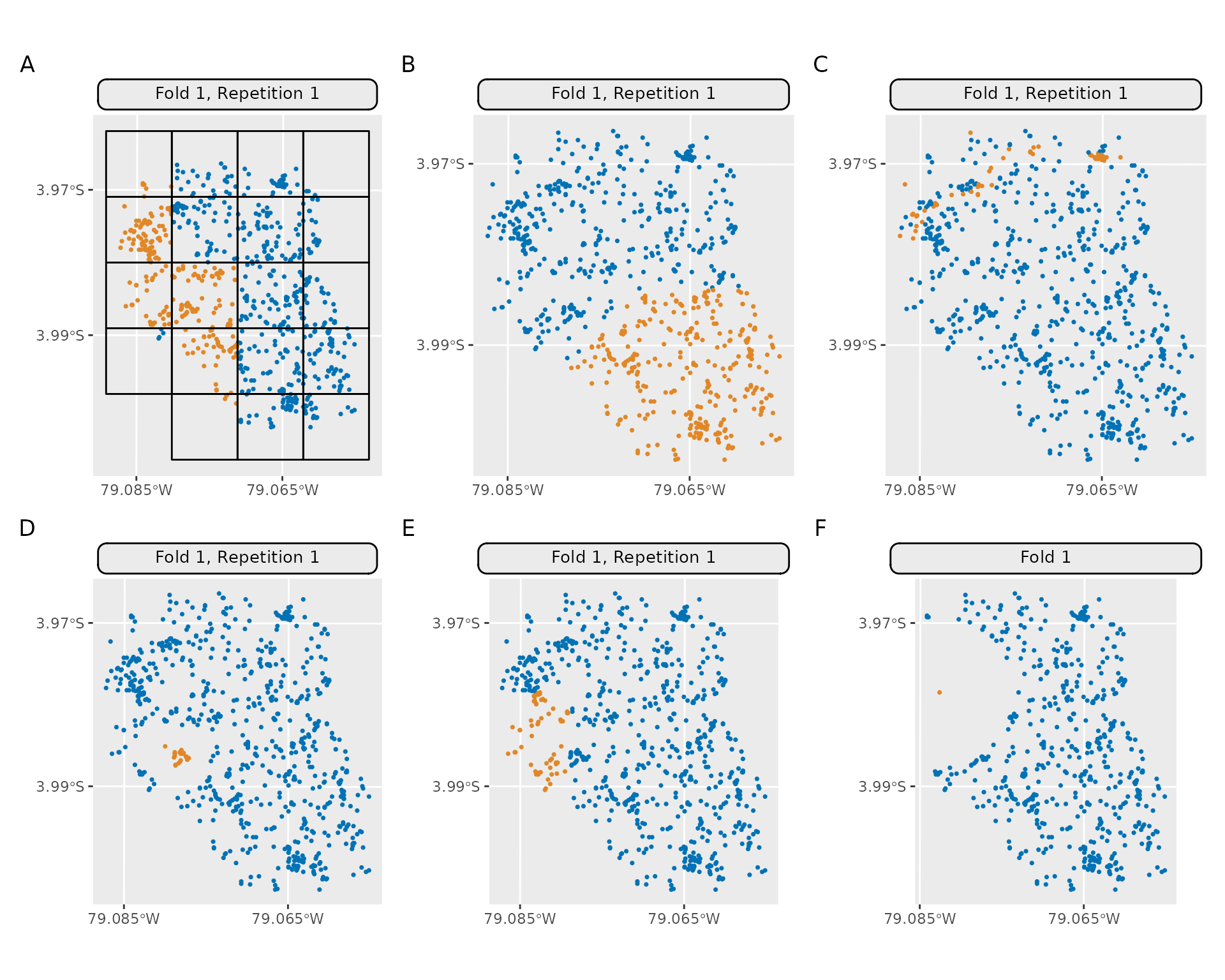## 3D Visualization (spatiotemporal)

{mlr3spatiotempcv} makes use of {plotly} to create the 3D plots for visualizing spatiotemporal folds created via the CLUTO algorithm. Arranging multiple 3D plots in {plotly} is done via 3D subplots.

Unfortunately, {plotly}’s subplot implementation is not dynamic. This means that multiple “scene” objects need to be specified in plotly::layout() to determine the coordinates of the respective subplot. Depending on the number of chosen folds by the user in autoplot(), a different number of scenes with different coordinates needs to be given to align the plots properly.

Hence, manual action is needed to create a properly aligned grid of 3D plots.

Below is an example how to create a 2x2 grid showing four folds as 3D subplots. It makes use of the returned 3D plotly objects which are returned in a list by autoplot():

library(mlr3)
library(mlr3spatiotempcv)
resampling = rsmp("sptcv_cstf",
folds = 5, time_var = "Date",
space_var = "SOURCEID")

pl = autoplot(resampling, task_st, c(1, 2, 3, 4),
crs = 4326, point_size = 3, axis_label_fontsize = 10,
plot3D = TRUE
)

# Warnings can be ignored
pl_subplot = plotly::subplot(pl)

plotly::layout(pl_subplot,
title = "Individual Folds",
scene = list(
domain = list(x = c(0, 0.5), y = c(0.5, 1)),
aspectmode = "cube",
camera = list(eye = list(z = 2.5))
),
scene2 = list(
domain = list(x = c(0.5, 1), y = c(0.5, 1)),
aspectmode = "cube",
camera = list(eye = list(z = 2.5))
),
scene3 = list(
domain = list(x = c(0, 0.5), y = c(0, 0.5)),
aspectmode = "cube",
camera = list(eye = list(z = 2.5))
),
scene4 = list(
domain = list(x = c(0.5, 1), y = c(0, 0.5)),
aspectmode = "cube",
camera = list(eye = list(z = 2.5))
)
)Note: The image shown above is a static version created with plotly::orca().

Subplot titles can unfortunately not created dynamically. However, there is a manual workaround via annotations show in this RPubs post.

## Examples of spatial partitioning (2D)

The following plots are based on a three fold partitioning of the ecuador example task (expect method p_buffer which is LOO-CV). They do not claim to make sense or make use of useful settings - they only have a showcase purpose. The code which produced the plots can be found here.• A: "spcv_block"

• B: "spcv_coords"

• C: "spcv_env"

• D: "spcv_disc"

• E: "spcv_tiles"

• F: "spcv_buffer"